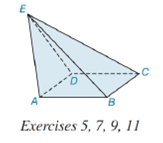Chapter 9.2, Problem 5EElementary Geometry For College St...

7th Edition
Alexander + 2 others
ISBN: 9781337614085

Solutions

Chapter
SectionElementary Geometry For College St...

7th Edition
Alexander + 2 others
ISBN: 9781337614085
Textbook Problem

In the solid shown, base A B C D is a square.a) Is the solid a prism or a pyramid?b) Name the vertex (apex) of the pyramid.c) Name the lateral edges.d) Name the lateral faces.e) Is the solid a regular solid?To determine

(a)

To name:

The solid in the given figure is a prism or a pyramid.

Explanation

Given:

The figure given below

and base ABCD is a square.

Properties Used:

A pyramid is made by connecting a base to an apex. The base is flat with straight edges, no curves, hence, a polygon and all other faces are triangles. The altitude/height of the pyramid is the line segment from the vertex perpendicular to the plane of the base.

To determine

(b)

To name:

The vertex in the given figure.

To determine

(c)

To name:

The lateral edges in the given figure.

To determine

(d)

To name:

The lateral faces in the given figure.

To determine

(e)

To find:

The given figure is a regular solid.

Still sussing out bartleby?

Check out a sample textbook solution.

See a sample solution

The Solution to Your Study Problems

Bartleby provides explanations to thousands of textbook problems written by our experts, many with advanced degrees!

Get Started

In problems 49-54, rationalize the denominator and then simpify. 49.

Mathematical Applications for the Management, Life, and Social Sciences

In Exercises 1-6, show the interval on a number line. 5. (0, )

Applied Calculus for the Managerial, Life, and Social Sciences: A Brief Approach

Expand each expression in Exercises 122. (x22x+1)2

Finite Mathematics and Applied Calculus (MindTap Course List)

Find the sum of the series. 29. n=1[tan1(n+1)tan1n]

Single Variable Calculus: Early Transcendentals